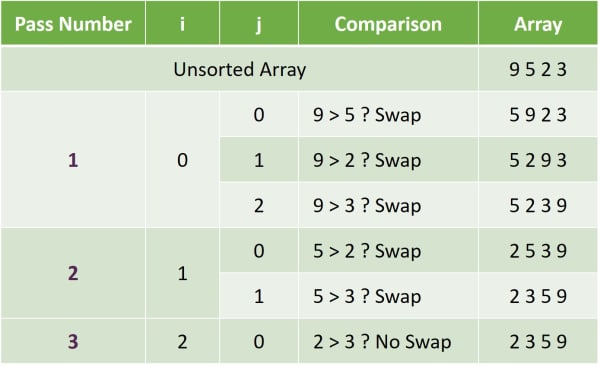# Bubble Sort

## ICSE Computer Applications

Sorting means arranging the elements of the array in either ascending or descending order.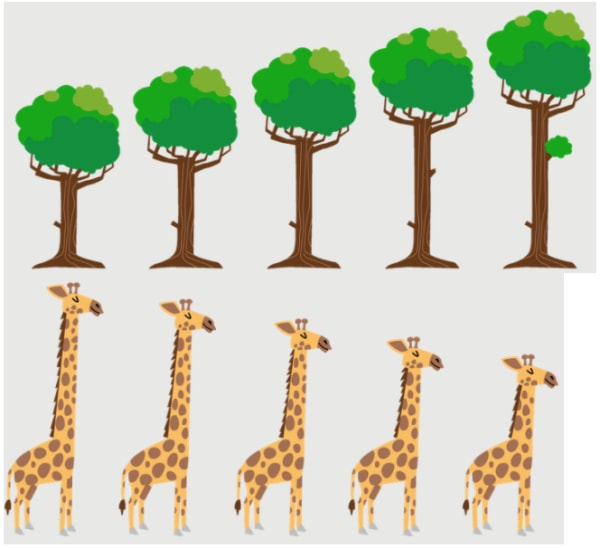When things are sorted, it makes it easier for us to find them quickly. We saw this in Binary Search which works on sorted arrays and can find the elements much faster than Linear Search.

In programming, there are multiple ways for sorting. ICSE Computer Applications syllabus prescribes two of them:

1. Bubble Sort
2. Selection Sort

## Sorting Playing Cards With Bubble Sort

Let’s keep programming and Java aside for a while and try to understand Bubble Sort through a simple real-world example.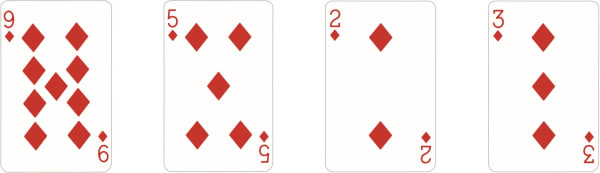I have these four cards and I want to arrange them in ascending order using Bubble Sort method. After sorting, card two should be the first card followed by card three then card five and nine should be the last card.

In Bubble Sort, we compare adjacent elements and swap them if they are in the wrong order.

#### First Pass

To sort these cards using Bubble Sort, we will compare the first 2 cards. As, the first card nine is greater than the second card five so they are in wrong order. Remember, we need to arrange these cards in ascending order and these two cards are not in ascending order so we will swap them.

Next, we will compare nine with two. As the cards are in wrong order, we will swap them.

After that, we will compare nine with three. Again, the cards are in wrong order, let’s swap them.

We have gone through all the cards once. We call this a pass. We can say that we have completed first pass of sorting the cards.

Notice that at the end of this first pass, the last card is in its correct position. The largest of these four cards is nine and at the end of first pass it is correctly placed at the last position.

#### Second Pass

We will start our second pass through these cards. We will compare five and two. We will swap them as five is greater than two. Next, we will compare five and three. Cards are in wrong order so let’s swap them. With this our second pass completes and the last two cards are placed in their correct positions.

#### Third Pass

We will start our third pass to sort the remaining two cards. As two is less than three, we will not swap them. With this our third pass also completes and now our cards are sorted in ascending order.

You might wonder why the third pass was required as the cards were sorted at the end of second pass itself. Keep in mind, you and I as humans can look at the cards and tell that they are in ascending order, but a computer does not have this luxury. It needs to go through the cards and check if any swap is required or not. I hope this example gave you some understanding about Bubble Sort.

To summarize, in Bubble Sort we repeatedly compare adjacent elements and swap them if they are in wrong order. Bubble Sort is a simpler algorithm to learn in that its steps can be translated into code one to one.

## Bubble Sort Java Program

```public class KboatBubbleSort
{
public static void displayArray(int arr[]) {
for (int i = 0; i < arr.length; i++) {
System.out.print(arr[i] + " ");
}
System.out.println();
}

public static void main(String args[]) {
int arr[] = {9, 5, 2, 3};
int n = arr.length;

//Bubble Sort
for (int i = 0; i < n - 1; i++) {
System.out.println("\nPass Number: " + (i + 1));
for (int j = 0; j < n - i - 1; j++) {
System.out.println("\nComparing "+ arr[j]
+ " and " + arr[j+1]);
if (arr[j] > arr[j + 1]) {
System.out.println(arr[j]
+ " is greater than "
+ arr[j + 1]);
System.out.println("Swapping "
+ arr[j] + " and "
+ arr[j + 1]);
int t = arr[j];
arr[j] = arr[j+1];
arr[j+1] = t;
System.out.println("Array after swap: ");
displayArray(arr);
}
}
}

System.out.println("\nSorted Array:");
displayArray(arr);
}
}```

#### Output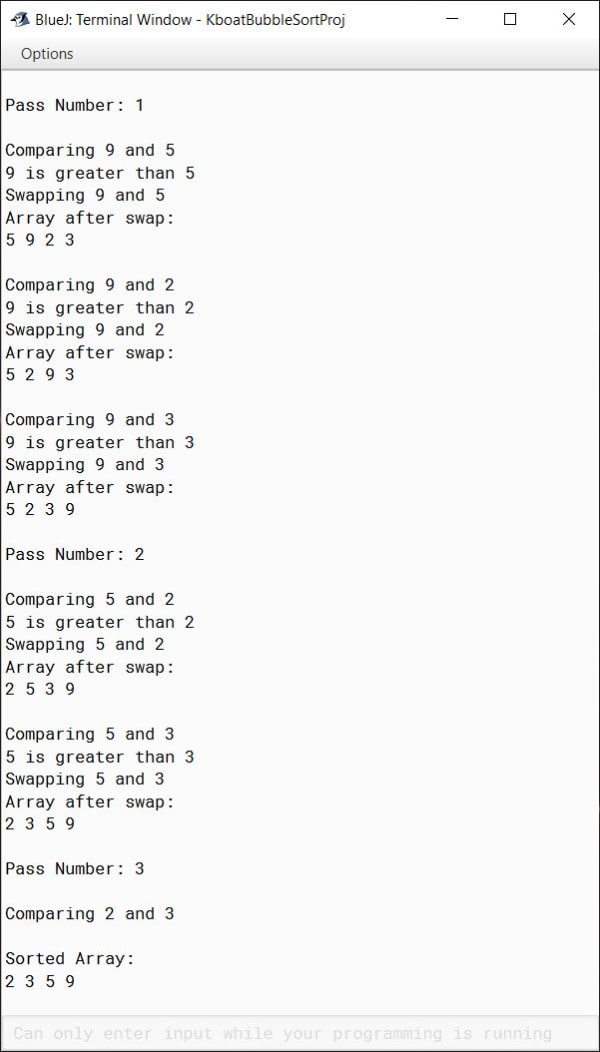The `displayArray` method is a very simple method for printing the elements of the array. It takes an int array `arr` as its argument and in the loop, it prints all the elements of the array. This method along with the additional print statements are added to the program so that we can get a good detailed output. It will help us understand what is going on in each step of the sorting process. As you can see in the output of the program above, at each step the program is printing what it is doing. Once we have gone through the program, I will remove these print statements and show you the program to give just the sorted array as the output. Let's continue with the program.

```int arr[] = {9, 5, 2, 3};
int n = arr.length;```

Coming to the main method, in the first two lines shown above, we declare and initialize an int array with nine, five, two and three as its elements. Then we store the length of this array in the variable n.

```//Bubble Sort
for (int i = 0; i < n - 1; i++) {
System.out.println("\nPass Number: " + (i + 1));
for (int j = 0; j < n - i - 1; j++) {
System.out.println("\nComparing "+ arr[j]
+ " and " + arr[j+1]);
if (arr[j] > arr[j + 1]) {
System.out.println(arr[j]
+ " is greater than "
+ arr[j + 1]);
System.out.println("Swapping "
+ arr[j] + " and "
+ arr[j + 1]);
int t = arr[j];
arr[j] = arr[j+1];
arr[j+1] = t;
System.out.println("Array after swap: ");
displayArray(arr);
}
}
}```

After that, we come to the Bubble Sort logic shown above. We need to make multiple passes through the array and in each pass, we will compare adjacent elements. To do this, we use two nested for loops. The outer loop controls the number of passes we make through the array. To sort an array of size n, we need to make n – 1 number of passes through the array. Size of our array `arr` is 4 so we need to make 3 passes through `arr` to sort it.

In the inner loop, we compare adjacent elements and swap them if they are in wrong order. Recall from our playing cards example that in each pass we place one element of the array in its correct position. In the first pass, we place the last element of the array in its correct position, so we have sorted one element. In the second pass we place second last element in its correct position, so we have sorted two elements and so on. So, at the end of each pass we have sorted the same number of elements as the number of that pass. The number of elements that we need to sort keeps reducing with each pass. We translate this into our inner for loop by setting its condition as `j < n – i – 1`. `i` gives the number of completed passes. By subtracting it like this, we are reducing the number of elements to compare by the number of elements we have already sorted. As we want to sort the array in ascending order, we check if first element is greater than second and swap them if the condition tests true.

Let’s go over the program line by line now and try to understand how it will sort this array `arr`. As I already mentioned, in the first two statements, `arr` is initialized and `n` gets a value of 4 which is the size of `arr`. Sorting begins with the first iteration of the outer for loop. `i` becomes 0. Pass number 1 is printed to the console. Then the inner for loop starts. `j` is initialized to zero. Condition is true as `j` is less than 3. 9 is compared to 5. The if check returns true so 9 and 5 are swapped in the array. We use `displayArray` method to print the current state of array to the console. Program control goes back to the beginning of the inner for loop. `j` becomes 1. Condition is true so program control enters the loop. 9 and 2 are compared. As 9 is greater than 2, they are swapped. Program control again goes back to the beginning of inner for. `j` is incremented to 2. Condition is true, program control enters the loop. 9 and 3 are compared. As 9 is greater than 3, they are swapped. Program control again goes back to the beginning of inner for. `j` is incremented to 3. This time the condition tests false. Inner loop is not executed, and program control jumps to the end of outer for. First pass through the array is completed and 9 is placed in its correct position.

Program control again goes back to the beginning of outer for loop. `i` is incremented to 1. Second pass through the array starts. `j` is initialized to 0. Notice that for the second pass, condition of inner for is `j` less than 2 whereas for the first pass it was `j` less than 3. We have reduced the number of elements to sort by the number of passes already completed. Condition of inner for is true, 5 and 2 are compared and swapped. In the next iteration of inner for, `j` becomes 1. 5 and 3 are compared and swapped. Second pass through the array is completed, 5 and 9 are now sorted.

`i` becomes 2 and third pass begins. `j` is initialized to 0. This time the condition is `j < 1` so inner for loop will execute only once. And that is all we need also as we just need to check two more elements for the array to be completely sorted. 2 and 3 are compared. As 2 is less than 3, they are already in ascending order, no need to swap them. Program control goes back to beginning of inner for, `j` is incremented to 1. Condition becomes false and program control comes to the end of outer for.

It goes back to the beginning of outer for and `i` is incremented to 3. Condition of outer for becomes false. Program control jumps to the println statement after the outer for and displays the array by calling `displayArray` method.

The array is now completely sorted. And we reach to the end of Bubble Sort program.

In the beginning I said that I will show you the program without the extra println statements and displayArray method. Here it is:

```public class KboatBubbleSort
{
public static void main(String args[]) {
int arr[] = {9, 5, 2, 3};
int n = arr.length;

//Bubble Sort
for (int i = 0; i < n - 1; i++) {
for (int j = 0; j < n - i - 1; j++) {
if (arr[j] > arr[j + 1]) {
int t = arr[j];
arr[j] = arr[j+1];
arr[j+1] = t;
}
}
}

System.out.println("Sorted Array:");
for (int i = 0; i < n; i++) {
System.out.print(arr[i] + " ");
}
}
}```

#### Output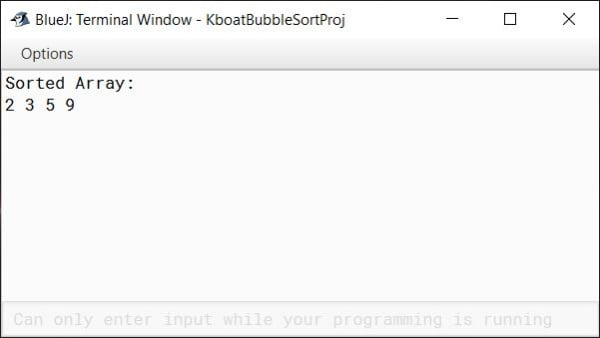The below table summarizes all the steps involved in sorting the array `arr` that I explained just now. You can use this as a reference to revise Bubble Sort.